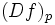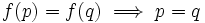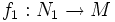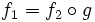# Submanifold (differential sense)

Let$M$ be a differential manifold. A submanifold of$M$ can be viewed as the following data: An abstract differential manifold$N$ along with a smooth map$f$ from$N$ to$M$ such that:
1. The map$f$ is an immersion; in other words, the induced map$(Df)_p$ on the tangent space at any point$p \in M$ is injective
2. The map is injective i.e.$f(p) = f(q) \implies p = q$
Note that when$N$ is compact, the third condition is redundant, because any injective map from a compact space to a Hausdorff space is an embedding.
To complete the definition, we need to observe that a submanifold is completely determined, upto diffeomorphism, by its set-theoretic image in the manifold. In other words, if$f_1:N_1 \to M$ and$f_2:N_2 \to M$ are submanifolds with the same set-theoretic image, then there is a diffeomorphism$g:N_1 \to N_2$ such that$f_1 = f_2 \circ g$.